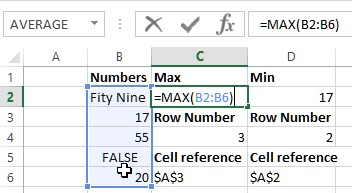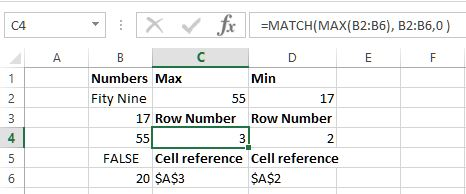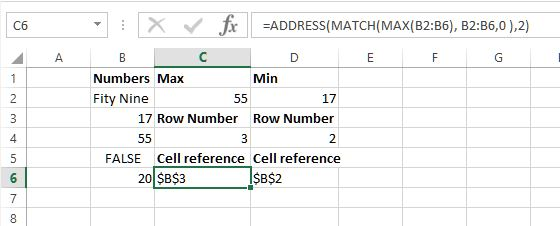# Max & Min value in Excel

##### How to locate maximum and minimum value in Excel cell range?

In previous post, we learnt about VLOOKUP and HLOOKUP function in Excel. In this post, we shall learn about MAX, MIN, MATCH and ADDRESS function of Excel.

### MAX function

MAX function in Excel is used to return the larget value in the set of values. It ignores the textual or logical values.

In C2 cell, the formula is '=MAX(B2:B6)' that gets the maximum values between B2 to B6 by ignoring any textual and logical values.The result is below.### MIN function

MIN function in Excel is used to return the smallest value in the set of values. It ignores the textual or logical values.

In D2 cell, the formula is '=MIN(B2:B6)' that gets the minimum values between B2 to B6 by ignoring any textual and logical values and the result is below.### Getting the relative position of a value using MATCH function

To get the relative position of the maximum value withing given range, we can use MATCH function.

In below example, C4 cell contains '=MATCH(MAX(B2:B6), B2:B6,0 )' formula that does following

1. 1st parameter gives the maximum value withing B2 to B6
2. 2nd parameter specifies the range within which to match the value
3. 3rd parameter specified whether to find exact value, or less than or greater than value.
As the mximum value is 55 that is coming at the 3rd position in the range so we get 3 in C4.### Getting the cell reference of maximum value using ADDRESS function

To get the cell reference of the maximum value within the given range, we can use ADDRESS function.

In C6 cell, we have '=ADDRESS(MATCH(MAX(B2:B6), B2:B6,0 ),2)' formula that does following

1. The 1st parameter gets and Maximum value and then its position
2. 2nd parameter specifies column number
and the result we get is \$B\$3.Views: 4195 | Post Order: 32